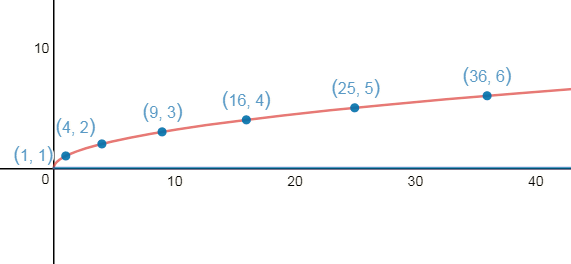## Monday, October 23, 2017

### Diophantine Desmos

It may happen that you have a real valued function, but only want to find those points where both the input and output are integers.

For example, consider y = sqrt(x). Suppose you had a graph of sqrt(x) and wanted to show which values of x give an integer result for y, i.e. which values of x are perfect squares:y = sqrt(x) with only integer solutions shown

I recently learned how you can find and display integer solutions for certain types of equations in Desmos, and thought I would write a post about how to do this.

When we care only about the integer solutions of an equation, we refer to it as a Diophantine equation. In general, Diophantine equations can take many forms - the technique described here can be used in a limited class of problems where you can express your Diophantine equation as a function k = f(n), where f takes integers n as inputs and want to know which outputs k are also integers (k > 0).

step 1 - define your real valued function
Let's see how to apply this method using sqrt(x). First, plotting the real-valued f(x) = sqrt(x) function, and creating a list of points by evaluating it for a range of integers, shows there are many points on the graph where we put in an integer, and get out a non-integer.

What we do next is to use some of the functions built into Desmos to create an integer detector function, that we can apply to the outputs of f(x).

step 2 - create the integer detector function
We'd like to build a function that can tell if a given input is an integer or not, and then use this to find out when we have an integer value for f(n).

We would like to create an indicator function for integers that behaves like this:

To do this, we can make use of some built in functions in Desmos - the ceiling and floor functions.

The function ceil rounds a decimal value up to the nearest integer, and floor rounds it down to the nearest integer.  Consequently, floor(x) <= ceil(x), with equality only if  x is an integer. Note also that for x > 0,  ceil(x) != 0, and floor(x)/ceil(x) <= 1, with equality only if x is an integer. The function floor(x)/ceil(x) is almost what we want - it is equal to 1 only when x is an integer. To get a function that is zero when x is not an integer, we take the floor again:

By composing t and f, we obtain a function tf which tells us when the values of f are integers. Plotting t(f(x)) for a range of integer inputs shows us which values of f give integer results.

points in red show values for (x, tf(x)), ponts
in blue show values for (x, f(x)).

The final step is to integrate tf into our graph so that we can plot the integer solutions to y=f(x).

step 3 - taking advantage of undefined
Desmos handles undefined points gracefully - it just will not plot them. Consequently, if we create a function g, which is identical to f where f takes on integer solutions, but is undefined when it does not, we can get Desmos to plot exactly the points we want and no others.

To create g, we can combine f with our indicator function t:

Plotting points using this new function shows only those which correspond to integer solutions for f(x).

integer solutions to y = sqrt(x), graph here

Another example - Pythagorean triples
We encounter another example of Diophantine equations when trying to find Pythagorean triples. A Pythagorean triple is an integer solution to the equation a^2 + b^2 = c^2.

At first it might look like we can’t apply the method developed above to the problem of finding Pythagorean triples - there seem to be too many variables in play. However, we can explore the problem within certain ranges by taking advantage of other features that Desmos provides.

We can first define our function as a two variable function, and then use partial evaluation to obtain a new single variable function, parameterized by a slider p.

This allows us to explore Pythagorean triples where one of the legs of the triangle is fixed (determined by the value p). Using the method described above, we can find triples involving p as one of the legs.

some triples found by Desmos,
where p=24 (graph here)

For example, with p = 24, Desmos finds the triples (24, 7, 25), (24, 18, 30), and others shown in the image above.

Some older Desmos-related posts:
- Brain and Propeller Fractals in Desmos
- Polygonal Number Diagrams using Desmos
- Spirals in Desmos
- Spirolaterals in Desmos